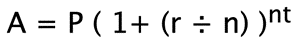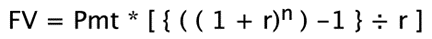# How To Calculate the Interest Rate for Your Savings Account

We are committed to sharing unbiased reviews. Some of the links on our site are from our partners who compensate us. Read our editorial guidelines and advertising disclosure.

Having money in the bank isn’t just about convenience—it’s also an investment. But is it a good investment?

To answer this question, you need to know how to calculate interest rates. Maybe you want to see how much your current savings account is making you or you want to compare savings account interest rates over time.

Either way, here’s what you need to know to do your calculations properly.## Calculating simple interest

Your interest is calculated by factoring the amount of money in your account with the interest rate and the amount of time the amount has been in the account.

Most savings accounts offer a yearly interest rate. That means you’ll make the stipulated amount after the money has been in your account for a year.

Here’s the equation to calculate simple interest:

• Interest = Principal x Rate x Number of Periods

The principal is the beginning balance, the interest rate is the given rate expressed over a certain period (usually a year), and the number of periods is the number of times the full duration has elapsed.

So, if you put \$100,000 into a bank account with a 1% interest rate for 2 years, the equation would look like this:

• 100,000 x 0.01 x 2 = 2000

This equation is the simplest way to calculate basic interest. The reality of how most savings accounts are used often lends itself to more complicated calculations. Still, if you’re looking for a simple analysis of what basic interest rates look like over a given period of time, this is a useful equation.

One thing to keep in mind is that the current average savings account interest rate is around 0.13%. You can find savings accounts with much higher interest rates, but you have to know where to look.

## Calculating compounding interest

Interest can compound depending on how often interest payments are made. For example, if you get paid interest payments every month, your principal will increase monthly. So your yearly interest will also increase.

The equation below shows you how to calculate compound interest:The variables are:

• A = total future value
• P = principal deposit
• r = interest rate
• n = number of compounding periods
• t = number of periods that have passed or will pass

Once you know your numbers for the variables, you simply plug them into the equation. Here’s an example of how to use this equation to calculate compounding interest:

1. A = \$10,000 [ 1 + (.01 ÷ 12) ] 12 x 1
2. A = \$10,000 [ 1 + ( .0.0008334 ) ] 12
3. A = \$10.000 [ 1.0008334 ] 12
4. A = \$10.000 [ 1.1.01004676825 ]
5. A = \$10,100.47

That’s a total of \$100.47 of compounded interest over twelve compounding periods. This calculation shows a more complete picture of your interest payments over time.

## Calculating interest with regular deposits

Both equations above show you how to calculate interest given a single lump sum principal. But if you’re planning to pay into your savings account monthly, your calculations will need to be a bit more complicated.

Here’s what the equation looks like:The variables are:

• FV = future value of your account
• Pmt = monthly payment amount
• r = monthly interest rate (be sure to divide annual rate by 12)
• n = number of months

Using this equation, you can calculate your interest rate based on monthly payments and get an even more accurate picture of what using a given savings account will look like over time.

## How to earn more interest from your savings account

Now that you’re equipped with the mathematical tools you need to calculate interest rates, it’s time to consider what to do next. Perhaps you’re looking for a high-yield savings account that can compound your interest fast.

Well, you’re in luck. There are many competitors in the banking market with relatively high interest rates. Small Business Bank and Lili, for example, offer interest rates as high as 1%.

While a savings account will never be the most lucrative investment option, it’s a safe place to store your money. Savings accounts ensure your cash is available when you need it and that you’re earning a little interest in the meantime.Get a better business bank account

Want affordable banking with great perks? With Bluevine, you can get a fee-free business checking account―and you can even earn up to \$5,000 in interest.

## The takeaway

Calculating interest is a simple mathematical procedure. You can calculate simple interest, compound interest, and interest that accrues over multiple months with multiple deposits. Once you know the equations, you’re ready to start comparing savings accounts for maximum dividends.

In fact, we recently compared some of the highest yield savings accounts on the market today.

### Related content

Disclaimer

At Business.org, our research is meant to offer general product and service recommendations. We don't guarantee that our suggestions will work best for each individual or business, so consider your unique needs when choosing products and services.Written by
Andrew Mosteller